• WORD格式 专业资料整理 MATLAB作业 方法一 N=200;dt=0.001;n=1:200; x=3*sin(2*pi*10*n*dt)+3*sin(2*pi*30*n*dt)+sin(2*pi*40*n*dt)+sin(2 *pi*50*n*dt)+sin(2*pi*60*n*dt)+6*sin(2*pi*80*n*dt; %建立时间序列 X=...
• 今天，二狗给大家讲一讲Matlab实现傅里叶变换。大家都知道，信号分为两种，确定信号和不确定信号。在确定信号，有两个非常重要的类别，时域分析和频域分析。而将两者充分结合的，就是我们今天要讲的傅里叶变换。绝...


嗨，大家好。二狗又回来了。今天，二狗给大家讲一讲Matlab实现傅里叶变换。大家都知道，信号分为两种，确定信号和不确定信号。在确定信号中，有两个非常重要的类别，时域分析和频域分析。而将两者充分结合的，就是我们今天要讲的傅里叶变换。绝大多数工科狗在大一或者大二的时候，都或多或少接触过傅里叶变换。二狗也不例外。当初二狗学《复变函数与积分变换》时，差点被搞成死狗，就是因为傅里叶变换。下面这个三维图就是频域和时域上的周期函数，非常形象的显示了这个道理。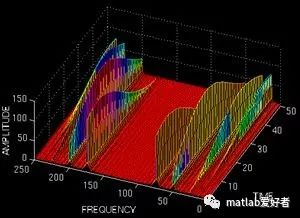由上可知，在空间中。从频率或者时域的角度都可以描述这个周期函数。下面，我们先帮助大家简单回忆一下傅里叶变换的基本原理。周期函数都是时域表达式，既然有周期，当然也就有频率。有些时候，我们需要用到函数的频域表达式。这就需要在频域和时域之间转化。最简单的就是利用周期函数的三角函数展开式，公式如下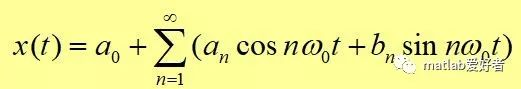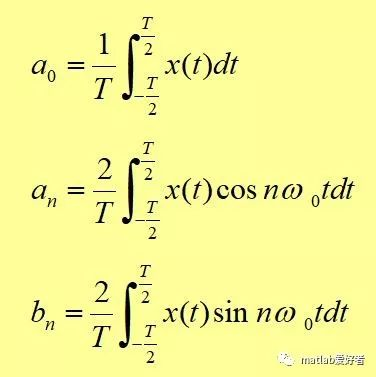以上是三角展开形式，今天我们不用这个，完全是为了方便大家理解，所以先说了一个简单的(见谅)。我们今天要用的是复指数形式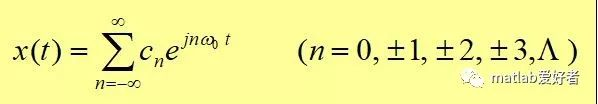当然，两种形式可以相互转换的，只需要利用欧拉公式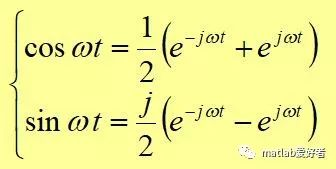下面，我举一个简单的函数作为例子f(t)=sin(t)+2*cos(t)。我们来对它进行傅里叶变换。根据已有的公式，我们一步步推进，我们先画出这个函数的图像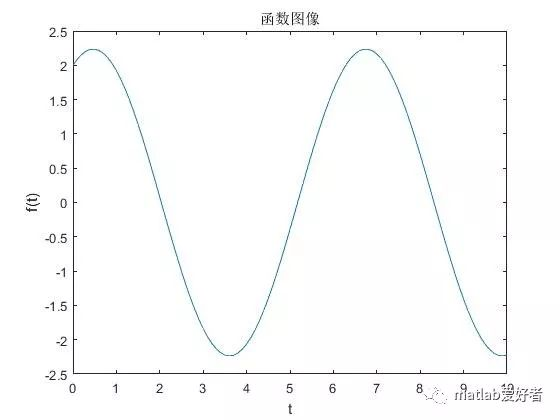下面的几步将傅里叶变换的参数全部求解出来，依据的就是复指数形式表达式(看不懂的同学倒回去看一看刚才讲的公式)。k=-N:N;W=pi*k/(N*dt);Fw=dt*ft*exp(-j*t1'*W);然后，对Fw进行一次绝对值变化。得到最终的结果。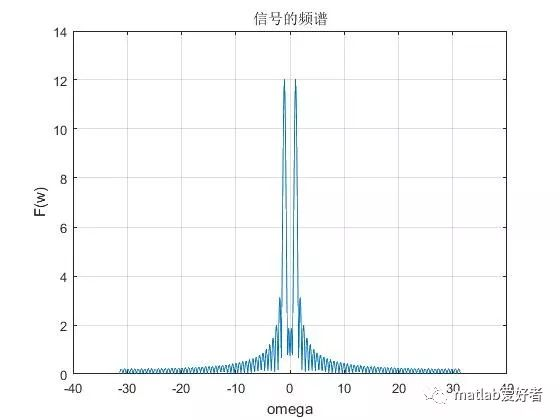以上就是信号的频域分析。即傅里叶表达式图像既然有频域函数，那当然就有频谱图。周期函数往往是连续的，但是频率却是离散的。废话不说了，上代码：Ts=0.4;  %采样周期,可通过改变采样周期大小观察抽样信号的频谱变化dt=0.1;t1=0:dt:10;ft=sin(t1)+2*cos(t1);%subplot(1)plot(t1,ft);%gridaxis('auto');xlabel('t');ylabel('f(t)');title('函数图像');hold onfigureN=400;k=-N:N;W=pi*k/(N*dt);Fw=dt*ft*exp(-j*t1'*W);plot(W,abs(Fw));grid;axis('auto')xlabel('omega');ylabel('F(w)');title('信号的频谱');hold onfigure%下面代表抽样过程t2=0:Ts:10;fst=sin(t2)+2*cos(t2);plot(t1,ft,'r*');hold onfigure%stem适合画火柴棍型图stem(t2,fst);gridaxis('auto')xlabel('t');ylabel('fs(t)');title('抽样后的信号');%hold offFsw=Ts*fst*exp(-j*t2'*W);%subplot(224)plot(W,abs(Fsw));gridaxis('auto')xlabel('omega');ylabel('Fs(w)');title('抽样信号的频谱');

展开全文• 一个MATLAB中傅里叶级数变换的函数 很多朋友在做傅里叶级数变换时，会发自己的matlab中并不带有傅里叶级数变换函数，这就需要我们自己编一个级数变换函数。在大学高数我们学过傅里叶级数的变换原理，就是下面的公式...
一个MATLAB中傅里叶级数变换的函数
很多朋友在做傅里叶级数变换时，会发自己的matlab中并不带有傅里叶级数变换函数，这就需要我们自己编一个级数变换函数。在大学高数我们学过傅里叶级数的变换原理，就是下面的公式了，方便大家理解后面的代码。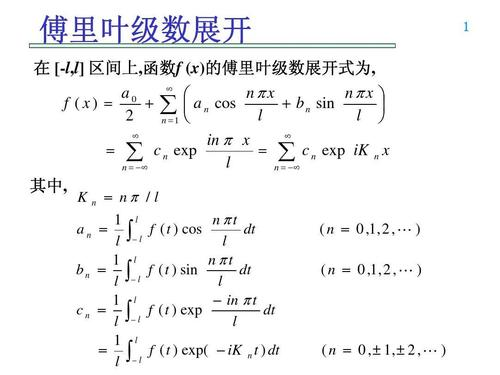具体的代码就是下面的了，大家要记得保存为fseries.m格式，今后用时直接调用就可以啦。

function [an,bn,f]=fseries(fx,x,n,a,b)
%傅里叶级数展开
%an为fourier余弦项系数
%bn为fourier正弦项系数
%f为展开表达式
%f为给定函数
%x为自变量
%n为展开系数
%a,b为x的区间,默认为[-pi,pi]

if nargin==3
a=-pi;
b=pi;
end
l=(b-a)/2;
if a+b
fx=subs(fx,x,x+l+a);
end
an=int(fx,x,-l,l)/l;
bn=[];
f=an/2;
for ii=1:n
ann=int(fx*cos(ii*pi*x/l),x,-l,l)/l;
bnn=int(fx*sin(ii*pi*x/l),x,-l,l)/l;
an=[an,ann];
bn=[bn,bnn];
f=f+ann*cos(ii*pi*x/l)+bnn*sin(ii*pi*x/l);
end
if a+b
f=subs(f,x,x-l-a);
end




展开全文• 傅里叶变换是一种线性积分变换，用于信号在时域（或空域）和频域之间的变换，在物理学和工程学有许多应用。1.傅里叶级数先从傅里叶级数讲起，任何周期函数都可以展开为正弦和余弦函数的和。周期为2 的 f(x), 能...
傅里叶变换是一种线性积分变换，用于信号在时域（或空域）和频域之间的变换，在物理学和工程学中有许多应用。1.傅里叶级数先从傅里叶级数讲起，任何周期函数都可以展开为正弦和余弦函数的和。周期为2的 f(x), 能展开成，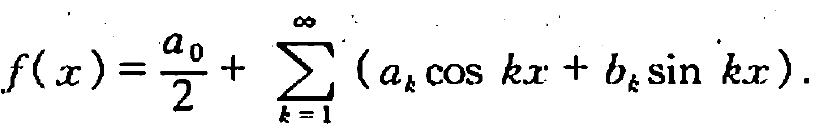或者写成其中Fn为复振幅。2. 连续傅里叶变换继续延申傅里叶级数的思想，计算连续傅里叶变换，即把用积分代替求和。连续傅里叶变换将可积函数 f(t) 成 复指数函数的积分或级数形式。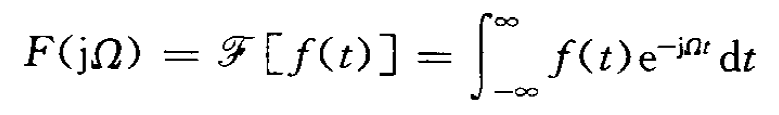傅里叶变换逆变换为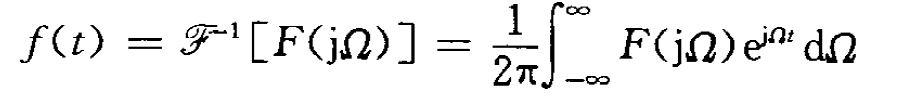代表角频率。3. 离散傅里叶变换类似的，离散时间信号x(n) 的离散傅里叶变换DTFT (Dicrete time Fourier transform)可以写为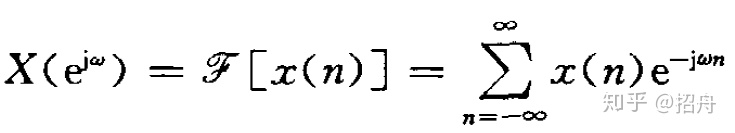逆变换为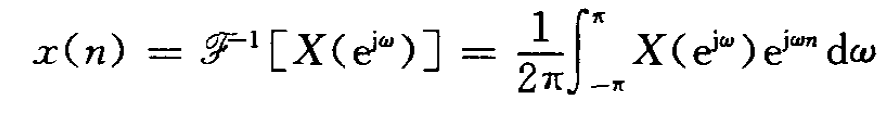表示频谱，一般为复数。注意DTFT中，时域是离散的，而频域依然是连续的。对DTFT频域离散采样之后的操作，称为离散傅里叶变换(DFT)。如果还不懂的话，可以看这个： 一幅图弄清DFT与DTFT,DFS的关系。一幅图弄清DFT与DTFT,DFS的关系 - BitArt - 博客园​www.cnblogs.com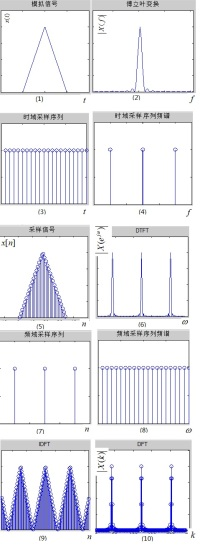4. 快速傅里叶变换在离散傅里叶变换(DFT)上，当N很大时，直接计算的方法，耗时太久。因此，有研究者提出了很多快速傅里叶变换(FFT)的算法。matlab实现快速傅里叶变换----clear;clcFs = 1000;            % 采样频率T = 1/Fs;             % 采样周期L = 1000;             % 信号长度   由此知，频率分辨率为 1hzt = (0:L-1)*T;        % 时间相量S = 0.7*sin(2*pi*50*t+pi/4) + sin(2*pi*150*t-pi/2);   % 原始函数Y = fft(S);P2 = abs(Y/L);  % 每个量除以数列长度 LP1 = P2(1:L/2+1);  % 取交流部分P1(2:end-1) = 2*P1(2:end-1); % 交流部分模值乘以2f = Fs*(0:(L/2))/L; %横坐标频率plot(f,P1)title('Single-Sided Amplitude Spectrum of S(t)')xlabel('f (Hz)')ylabel('|P1(f)|')----(引用自葛底斯堡：matlab之快速傅里叶变换（fft）​zhuanlan.zhihu.com得到结果如下：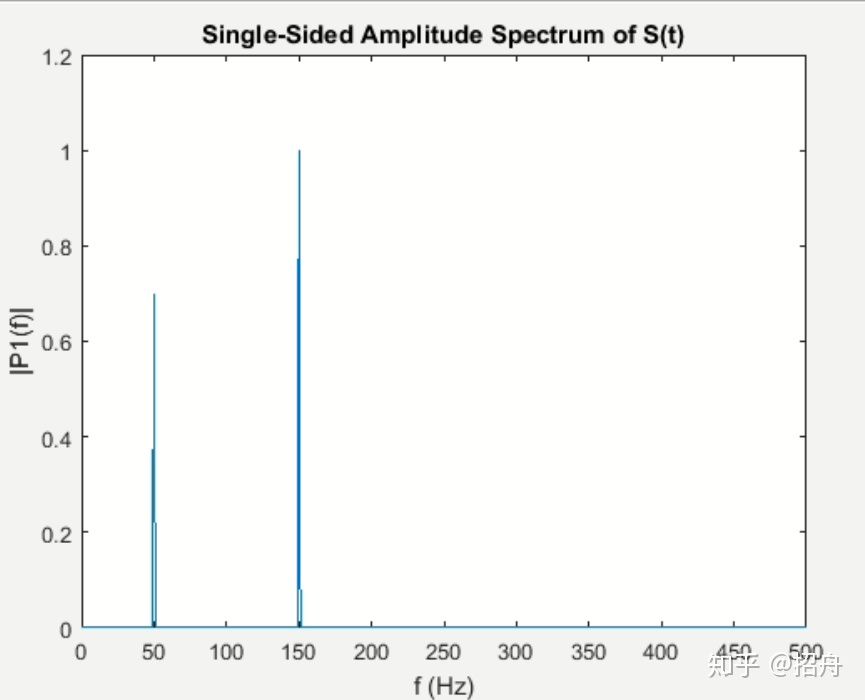特别要注意的有两点1). fft之后的实数值部分，并不是真的amplitude，还需要  abs(z1)/L*2;  2). 横坐标，频率的计算；5. 功率谱 (Power Spectral density, PSD)根据Weiner-Khintchine 定理，信号的功率谱和其自相关函数服从一对傅里叶变换公式，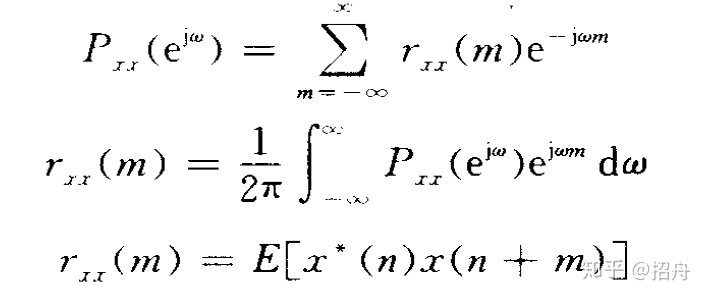但是实际计算中，会遇到两个问题 （a）不可能获得无限长度的信号 （b）有噪音。谱估计方法分为经典和现在方法。参考文献https://en.wikipedia.org/wiki/Fourier_transform 数字信号处理 姚天任https://blog.csdn.net/czyt1988/article/details/84995295
展开全文• 今天，二狗给大家讲一讲Matlab实现傅里叶变换。大家都知道，信号分为两种，确定信号和不确定信号。在确定信号，有两个非常重要的类别，时域分析和频域分析。而将两者充分结合的，就是我们今天要讲的傅里叶变换。绝...


嗨，大家好。二狗又回来了。今天，二狗给大家讲一讲Matlab实现傅里叶变换。大家都知道，信号分为两种，确定信号和不确定信号。在确定信号中，有两个非常重要的类别，时域分析和频域分析。而将两者充分结合的，就是我们今天要讲的傅里叶变换。绝大多数工科狗在大一或者大二的时候，都或多或少接触过傅里叶变换。二狗也不例外。当初二狗学《复变函数与积分变换》时，差点被搞成死狗，就是因为傅里叶变换。下面这个三维图就是频域和时域上的周期函数，非常形象的显示了这个道理。由上可知，在空间中。从频率或者时域的角度都可以描述这个周期函数。下面，我们先帮助大家简单回忆一下傅里叶变换的基本原理。周期函数都是时域表达式，既然有周期，当然也就有频率。有些时候，我们需要用到函数的频域表达式。这就需要在频域和时域之间转化。最简单的就是利用周期函数的三角函数展开式，公式如下以上是三角展开形式，今天我们不用这个，完全是为了方便大家理解，所以先说了一个简单的(见谅)。我们今天要用的是复指数形式当然，两种形式可以相互转换的，只需要利用欧拉公式下面，我举一个简单的函数作为例子f(t)=sin(t)+2*cos(t)。我们来对它进行傅里叶变换。根据已有的公式，我们一步步推进，我们先画出这个函数的图像下面的几步将傅里叶变换的参数全部求解出来，依据的就是复指数形式表达式(看不懂的同学倒回去看一看刚才讲的公式)。k=-N:N;W=pi*k/(N*dt);Fw=dt*ft*exp(-j*t1'*W);然后，对Fw进行一次绝对值变化。得到最终的结果。以上就是信号的频域分析。即傅里叶表达式图像既然有频域函数，那当然就有频谱图。周期函数往往是连续的，但是频率却是离散的。废话不说了，上代码：Ts=0.4;  %采样周期,可通过改变采样周期大小观察抽样信号的频谱变化dt=0.1;t1=0:dt:10;ft=sin(t1)+2*cos(t1);%subplot(1)plot(t1,ft);%gridaxis('auto');xlabel('t');ylabel('f(t)');title('函数图像');hold onfigureN=400;k=-N:N;W=pi*k/(N*dt);Fw=dt*ft*exp(-j*t1'*W);plot(W,abs(Fw));grid;axis('auto')xlabel('omega');ylabel('F(w)');title('信号的频谱');hold onfigure%下面代表抽样过程t2=0:Ts:10;fst=sin(t2)+2*cos(t2);plot(t1,ft,'r*');hold onfigure%stem适合画火柴棍型图stem(t2,fst);gridaxis('auto')xlabel('t');ylabel('fs(t)');title('抽样后的信号');%hold offFsw=Ts*fst*exp(-j*t2'*W);%subplot(224)plot(W,abs(Fsw));gridaxis('auto')xlabel('omega');ylabel('Fs(w)');title('抽样信号的频谱');

展开全文• 原文 定义： ﻿MATLAB帮助文件原文  ...​​1....式，y是序列，Y是序列的快速傅里叶变换。y可以是一向量或矩阵，若y为向量，则Y是y的FFT，并且与y具有相同的长度。若y为一矩阵，则Y是
• FFT（Fast Fourier Transformation）为一阶快速傅里叶变换函数，在数字信号处理有着广泛的应用，变换结果为复数。有些信号在时域上很难看出变化特征，但如果变换到频域之后，就很容易看出特征了。FFT把时域信号...fft
• 只分析一维度的 ...正变换： fftw_complex *in = fftw_malloc ( sizeof ( fftw_complex ) * n ); fftw_complex *out = fftw_malloc ( sizeof ( fftw_complex ) * n ); plan_forward = fftw_plan_dft_1d ...
• 可以使用 MATLAB傅里叶变换函数和反变换函数。 原理部分 低通滤波器指去除图像的高频成分，而低通滤波器指去除图像的高频成分。 考虑的有三种低通滤波器：理想滤波器、布特沃斯滤波器和高斯滤波器。 ...
• 1.这是通常情况下，输入一个多频率成分的函数，然后得到傅里叶变换clear显示结果：Fig 1 通常情况2. 这是在已有一串数据的情况下，想要对这串数据作傅里叶变换。clear显示结果：Fig 2 导入数据情况完成啦！...
• 1.这是通常情况下，输入一个多频率成分的函数，然后得到傅里叶变换clear;clc; Fs = 10000; % 采样频率，要大于时域信号最大频率的两倍 T = 1/Fs; % 采样周期，或者说是时域信号的时间步长，因为你处理的信号不会是...
• MATLAB中想要画一个sin函数是很容易的。比如:首先定义t = 0:0.01:10,然后画出y = sin(2*pi*t)就可以了，最多再加相角啊之类的参数。但如果在C语言中应该如何自己制造一个sin函数呢？应该借鉴数字信号处理里的抽样...
• MATLAB 是一套高性能的数值计算和可视化软件，它集 数值分析、矩阵计算、信号处理和图形显示于一体，构成了方 便、友好的用户界面环境。...processing) 工具箱函数，为信号与系统的分析提供了强有力 的工具。
• 在我使用神经网络进行音频信号分析时，我没有在TensorFlow找到可以处理音频的函数，这给我的项目进度带来了一些困难，所以我决定自己用TensorFlow来写一个音频信号短时傅里叶变换的脚本。在带有音频输入的...
• 模拟信号进行奈奎斯特采样后成为离散的数字信号，时域分析不太方便，通常要进行频域变换更好的分析信号，matlab中的fft和shiftfft两个函数可以快速的帮助我们进行频谱分析。两个函数存在一些差别。 关于fft的预备...fft
•   在Matlab中，做短时傅里叶变换需要使用函数spectrogram，而在Matlab2019中，引入了一个新的函数stft，下面我们就来看下这两个函数都如何使用。  短时傅里叶变换的基本原理就是将数据分段加窗，做fft，在分段时...
• 本文首发于i春秋,本人为本文原作者,转载请标明出处序言佛语有箴言：坐亦禅,行亦禅,一花一世界,一叶一如来世间万物是复杂的，但是又是纯粹的简单的，从宏观的花花世界到微观的原子电子，万物都在按照它的规律运行，...
• T2F函数，在matlab中傅里叶变换时调用,时间域信号转化为频率域
• 一种积分变换，它来源于函数的傅里叶积分表示。...傅里叶变换在物理学、电子类学科、数论、组合数学、信号处理、概率论、统计学、密码学、声学、光学、海洋学、结构动力学等领域都有着广泛的应用（例如在信号处理...
• （2）详细说明了MATLAB中fft函数的运用方法，并给出了fft幅度谱的求解代码。 但要真正了解快速傅里叶变换，核心是理解“FFT的计算原理”！！！ 目录 一、直观解释 二、本质原因之FFT的计算原理 （关键） 三、...
• [TFR,T,F]=TFRSTFT(X,T,N,H,TRACE) 　X : 信号. ...　H : 选择的平滑窗函数 (默认值: Hamming(N/4)). 　TRACE : 如果不为零，跟踪计算过程 (默认为 : 0). TFR : 得到的时频谱值（复数） 　F : 归一化...
• Matlab中，做短时傅里叶变换需要使用函数spectrogram，而在Matlab2019中，引入了一个新的函数stft，下面我们就来看下这两个函数都如何使用。 短时傅里叶变换的基本原理就是将数据分段加窗，做fft，在分段时会有...
• 参考资料：数学物理方法 （德）顾樵 编著这是我最近自学顾樵的《数学物理方法》的傅里叶级数和傅里叶变换两章时所做的笔记笔记写得很烂大家就随便看看吧笔记没有包含傅里叶变换的应用。这里还是应该稍微提一下。...
• MATLAB中自带的fft()函数可以帮助我们完成傅里叶变换的计算过程，不过直接调用fft()，画图之后发现横纵坐标的值和我们想的不一样，我们对下面这样的一个信号做fft Fs=1000; %采样率 N = 1; % 周期数 t=N; % 信号时长...信号处理
• MATLAB中可以用fourier函数来算出信号的傅里叶变换 syms w f(t) f(t)=sinc(t); F1 = fourier(f(t), t, w) ezplot(F1,[-10*pi,10*pi],1)
• 大纲从积分变换谈起积分变换的基本概念积分变换的来源傅里叶级数傅里叶级数的相关基础概念傅里叶级数的基石——三角函数系及其正交性三角函数系三角函数系的正交性傅里叶级数的含义傅里叶系数的导出傅里叶级数收敛...
• Matlab使用杂谈3-Fourier函数实现傅里叶变换傅里叶变换Matlab中的Fourier函数Fourier使用实例普通用法参数变换向量输入傅里叶变换无结果傅里叶逆变换 傅里叶变换 傅里叶展开式(Fourier expansion)是指用三角级数表示......

# matlab中傅里叶变换函数matlab 订阅